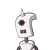# if the perimeter of a rectangle is 138 metres and the difference between the length and the breadth is 7 metres.what is the area o

if the perimeter of a rectangle is 138 metres and the difference between the length and the breadth is 7 metres.what is the area of the rectangle​

### 1 thought on “if the perimeter of a rectangle is 138 metres and the difference between the length and the breadth is 7 metres.what is the area o”

1.➸1178 square metres

explanation-

Let the length be x and breadth be y.

x – y = 7

The perimeter of rectangle is 138

2x + 2y = 138

x + y = 69

2x = 76

x = 38

y = 31

Area of rectangle = 31*38 = 1178

｡☆✼★━━━━━━━━━━━━★✼☆｡